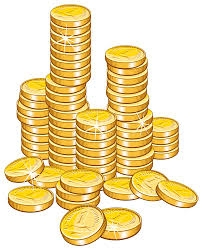Czech coins

John has 540 CZK, but he has 10 CZK coins and 20 CZK coins only. We know that he has 10CZK coins 7-times more than 20CZK coins. How many 10CZK coins and how many 20CZK coins did he save?

a =  42
b =  6

Step-by-step explanation:

10a+20b=540
a=7b

10•a+20•b=540
a=7•b

10a+20b = 540
a-7b = 0

a = 42
b = 6

Our linear equations calculator calculates it.Did you find an error or inaccuracy? Feel free to write us. Thank you!Tips to related online calculators
Do you have a system of equations and looking for calculator system of linear equations?